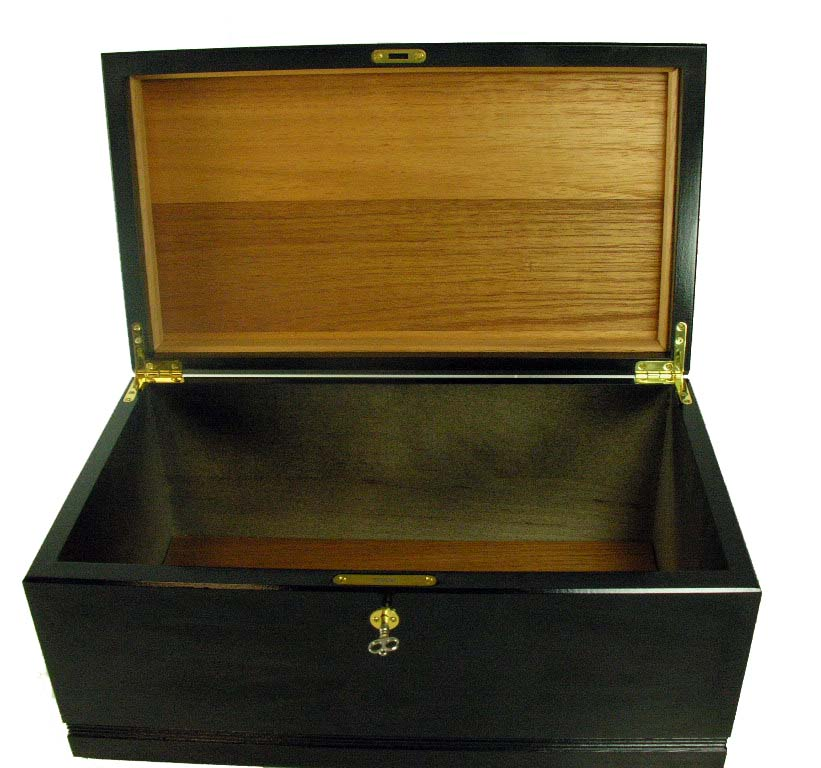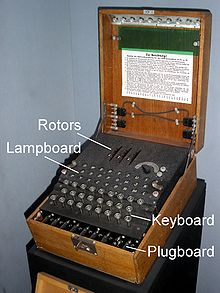#“不给力啊，老湿！”：RSA加密与破解

1. 云栖社区>
2. 博客>
3. 正文

## “不给力啊，老湿！”：RSA加密与破解

vamei 2013-12-19 22:31:00 浏览982

Eat the beancurd with the peanut. Taste like the ham.### RSA加密

A CHEF HIDE A BED

 A B C D E F G H I 1 2 3 4 5 6 7 8 9

A CHEF HIDE A BED

1 3856 8945 1 254

AGBEFBIDEAHED

（这里钥匙不小心和之前锁中的一个数字相同。这只是巧合。）

### 特工练习

1) 作为特工，用上面的算法为信息加密(你可能需要一些编程来计算，尝试用Python的数学计算功能？)。

2) 作为值班人员，验证143是钥匙，可以解密信息。

# By Vamei

#==== Agent ========
# coding covert: string to number
# By ASCII convention
def convert(original):
return map(ord, original)

# the input is a list of integers
def encrypt(input_list):
f = lambda x: (x**2987)%3937
return map(f, input_list)

# the input is the result of the encrypt function
def decrypt(encrypted_list):
f = lambda x: (x**143)%3937
return map(f, encrypted_list)

# convert numbers back to a string
def inv_convert(decrypt_list):
f = lambda x: str(unichr(x))
result = map(f, decrypt_list)
return "".join(result)

# Test
message = "Go to hell!"
secret = encrypt(convert(message))
print(secret)
public = inv_convert(decrypt(secret))
print(public)

### 费马与欧拉

1. 费马小定律

RSA的原理借助了数论中的“欧拉定理”(Euler's theorem)。17世纪的费马首先给出一个该定理的特殊形式，即“费马小定理”：

p是一个正的质数，a是任意一个不能被p整除的整数。那么，[$a^{p-1} - 1$]能被p整除。

*** 数学小贴士：

1) 除 (divide)，商余数：两个整数相除，有一个为整数的商，和一个余数。比如[$10/3 = 3, \,余1$]。我们用一个特别的方式记录这一叙述:

$$10 \equiv 1 (mod\, 3)$$

$$_3 = _3$$

$$[a]_n = [b]_n$$

$$a = nt + b$$

2) 整除 (divisible)：当一个整数a除以另一个整数b，余数为0时，那么我们说a可以被b整除。比如说，4可以被2整除。即

$$_2 = _2$$

3) 质数 (prime number)：一个质数是只能被[$\pm 1$]和这个数自身整除的整数（不包括[$\pm 1$]）。比如[$2，3，5，7，11，13$]等等。

******2. 欧拉定律

（这个传说的可信度不高，因为狄徳罗本人也是一位颇有造诣的数学家。）$$\phi(n) = 总数(从1到n-1，与n互质的整数)$$

*** “互质”的数学小贴士：

1) 因子 (factor)：每个整数都可以写成质数相乘的形式，每个这样的质数称为该整数的一个因子。

2) 互质 (relative prime)：如果两个整数没有公共因子，这两个质数互质。

******

m和n是互质的正整数。那么，[$\phi(mn) = \phi(m) \phi(n)$]        (2)

### RSA西游记

[$[ab]_n = [a]_n[b]_n$]        (3)$$[z^{\phi(n)}]_n = [z^k]_n = _n$$

$$[z^{de}]_n = [z^{kt + 1}]_n = [(z^k)^tz]_n = [z]_n$$

$$[z^{de}]_n = [z]_n$$

$$([z^e]_n)^d = [z]_n$$

(上面都默认余数是最小正余数，也就是说，10除以3的余数为1，而不是4。尽管4也可以算是10的余数，即[$_3 = _3$]。)3和8两个妖怪见到唐僧5，都被唠叨成了余数3。这样就观音姐姐就算法力无边，还是没法还原。为了让唐僧求余的时候，不会把数字弄混了，RSA算法要求所有妖怪z小于唐僧n。为了对足够多的字符转码加密，n必须大过最大的妖怪。### 总结

RSA算法是非对称算法。公开的加密方式，私有的解密方式。RSA安全的关键在于很难对一个大的整数进行因子分解。下一次，如果看到RSA被破解之类的消息，卧底必须大喊一声：“不给力呀，老湿！”